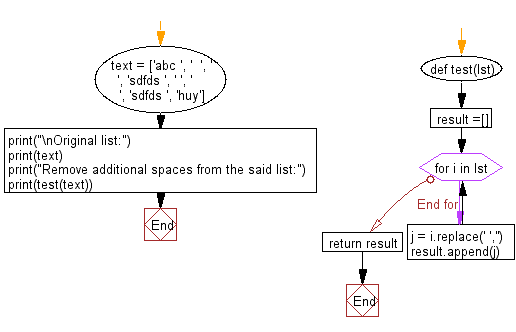﻿ Python: Remove additional spaces in a given list - w3resource# Python: Remove additional spaces in a given list

## Python List: Exercise - 206 with Solution

Write a Python program to remove additional spaces in a given list.

Sample Solution:

Python Code:

``````def test(lst):
result =[]
for i in lst:
j = i.replace(' ','')
result.append(j)
return result

text = ['abc ', '  ', ' ', 'sdfds ', ' ', '     ', 'sdfds ', 'huy']
print("\nOriginal list:")
print(text)
print("Remove additional spaces from the said list:")
print(test(text))
```
```

Sample Output:

```Original list:
['abc ', '  ', ' ', 'sdfds ', ' ', '     ', 'sdfds ', 'huy']
Remove additional spaces from the said list:
['abc', '', '', 'sdfds', '', '', 'sdfds', 'huy']
```

Flowchart:## Visualize Python code execution:

The following tool visualize what the computer is doing step-by-step as it executes the said program:

Python Code Editor:

Have another way to solve this solution? Contribute your code (and comments) through Disqus.

What is the difficulty level of this exercise?

Test your Python skills with w3resource's quiz

﻿

## Python: Tips of the Day

Floor Division:

When we speak of division we normally mean (/) float division operator, this will give a precise result in float format with decimals.

For a rounded integer result there is (//) floor division operator in Python. Floor division will only give integer results that are round numbers.

```print(1000 // 300)
print(1000 / 300)```

Output:

```3
3.3333333333333335```